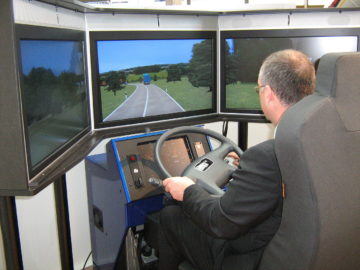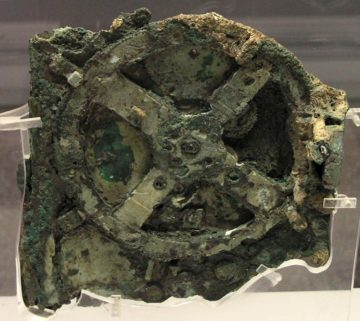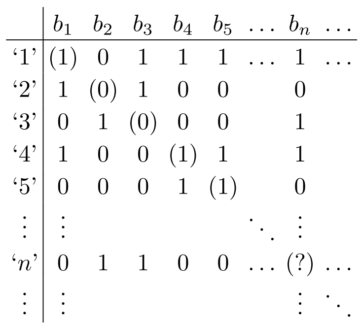# Gödel’s Proof and Einstein’s Dice: Undecidability in Mathematics and Physics – Part III

by Jochen SzangoliesThere are countless virtual realities, albeit as of yet, not exactly a replacement for the real thing. Image credit: wikimedia commons.

The simulation argument, most notably associated with the philosopher Nick Bostrom, asserts that given reasonable premises, the world we see around us is very likely not, in fact, the real world, but a simulation run on unfathomably powerful supercomputers. In a nutshell, the argument is that if humanity lives long enough to acquire the powers to perform such simulations, and if there is any interest in doing so at all—both reasonably plausible, given the fact that we’re in effect doing such simulations on the small scale millions of times per day—then the simulated realities greatly outnumber the ‘real’ realities (of which there is only one, barring multiversal shenanigans), and hence, every sentient being should expect their word to be simulated rather than real with overwhelming likelihood.

On the face of it, this idea seems like so many skeptical hypotheses, from Cartesian demons to brains in vats. But these claims occupy a kind of epistemic no man’s land: there may be no way to disprove them, but there is also no particular reason to believe them. One can thus quite rationally remain indifferent regarding them.

But Bostrom’s claim has teeth: if the reasoning is sound, then in fact, we do have compelling reasons to believe it to be true; hence, we ought to either accept it, or find flaw with it. Luckily, I believe that there is indeed good reason to reject the argument.

## Computers Can Do Almost NothingComputation has been with us a long time: the Antikythera mechanism dates from around the 1st century BC. Image credit: wikimedia commons.

Computation is all around us. Everything is smart: phones, watches, even homes and fridges. Hardly a week passes without a new machine learning model threatening what little is left of the idea of human uniqueness. We seem to live on a desert island whose shores are gradually eaten away by the rising tide of artificial intelligence. In short, it seems that there is nothing computers can’t do.

But in a technical sense, computers can hardly do anything at all. To make this point precise, we need to fix some notion of what, actually, a computer is. The classical working model goes back to the British mathematician Alan Turing, whose ‘A-Machines’ (nowadays simply called Turing machines) we have already met in the previous column in this series. To briefly recap, a Turing machine consists of a tape and a read/write head that can scan, delete, or draw new symbols onto it.

The intent of the Turing machine is to serve as an abstraction of what a human mathematician might do during the process of computation—manipulate symbols on a page. That Turing machines form an adequate model of computation is the content of the Church-Turing thesis, named after Turing and the American mathematician Alonzo Church, whose lambda-calculus provides an alternative formalization of computation that can be shown to be equivalent to that using Turing machines.

For simplicity, we may stipulate that our Turing machine only operates on the symbols ‘0’ and ‘1’. Thus, its operation is simply to write strings of bits onto the tape, and either finish at some point, or keep on doing so forever. A Turing machine might start with two numbers, encoded in binary, on its tape, and proceed to write their sum, then halt. But the bit strings might have more elaborate interpretations—they could encode color and coordinates of pixels on a screen, which might then show a certain image, which could represent one instant in some large-scale simulation of the universe, for instance.

But for our purposes, it suffices to think of these bit strings simply as numbers. Since we allow the machine to run indefinitely, we can think of it as creating, bit by bit, a real number, that may have an infinite and non-repeating decimal expansion. Now, a natural question arises: which numbers can be produced by a Turing machine? There are many numbers that are the output of some definite algorithm: numbers such as π, the ratio of a circle’s circumference to its diameter, or the golden ratio φ (Phi), or Euler’s constant e—all of these can be produced, to arbitrary accuracy, by a Turing machine chugging merrily along.

But there are limits to the machine’s power. Any Turing machine can be specified by some finite ‘recipe’ that list the symbols it operated upon, the internal states it can take on, and what it does if it reads a given symbol and is in a particular state. This set of instructions can be coded into a finite-length binary string—but a finite-length binary string can be interpreted as ordinary natural (or counting) number. Thus, we can give each possible Turing machine a finite serial number that identifies it uniquely.

Consequently, there are as many Turing machines as there are natural numbers. From this, we can immediately conclude that there are real numbers that no Turing machine can ever compute: thanks to Cantor’s theorem, we know that there are vastly more real numbers than natural numbers; hence, once all Turing machines are used up, there are still real numbers that none of them computes.

At first sight, this seems to be a counterintuitive idea: there are infinitely many natural numbers, so how can there be more real numbers? But the proof of that fact is surprisingly simple. For our purposes, we can look at infinite bit strings, which is after all what our Turing machines produce. Suppose we try the same trick with those we had applied to identify all Turing machines: assign them a serial number. Then, we can build up a list of bit strings, the first getting serial number ‘1’, the second ‘2’, and so on.Cantor’s diagonal argument: the nth bit of the nth number is inverted to yield a number that does not occur on the list.

Now suppose we build up a new bit string by taking the first bit of the bit string with serial number ‘1’, and inverting it, i.e. flipping its value; then taking the second bit of the bit string with serial number ‘2’, and inverting it again—and so on. The resulting bit string is different from any one already on our list: it differs from string ‘1’ (at least) in the first bit, from string ‘2’ in the second bit, and so on. Hence, our enumeration was incomplete!

But, no matter, you might say, we have infinitely many natural numbers for our enumeration, so let’s just add this one to the end. Except that this is no help: we can just use the same trick to generate another bit string not on the list.

Consequently, it isn’t possible to give each bit string a serial number: there are always more. But then, there are also more possible strings than there are Turing machines, since each of those does get a serial number. Hence, there must be bit strings no TM can produce.

In fact, we can also draw conclusions about the relative amount of bit strings that can and can’t be produced by a TM—i.e., computed. If you were to put the set of all bit strings into a hat, and randomly draw one, what is the probability that you get a computable one?

Again, the answer might seem surprising at first: the probability is exactly 0. Not very, very small, not tending to 0, but just 0, pure and simple. Conversely, the probability of drawing one that can’t be computed is exactly 1. Mathematicians speak of an event with probability 1 in such a case as occurring almost surely, and a probability 0 event as occurring almost never.

To illustrate this, suppose you’re throwing darts at a board, in such a way that you always hit the board, but it’s randomly distributed where you hit. The probability that it lands somewhere on that board is 1; the probability that it lands in the left half is 0.5, the probability that it lands in the top right quarter 0.25, and so on—the probability is simply given by the fraction of area.

But now consider a line, perhaps a diameter: since a line has area 0, the probability of hitting that line must also be zero. Moreover, consider hitting any given point: again, the probability is exactly 0. But one point, surely, will be hit—that’s why in this setting, probability 0 doesn’t mean impossible, and why mathematicians, being a rather circumspect sort, only speak of such things happening almost never.

Hence, the fraction of computable bit strings within all bit strings is like a point, or a line, within a finite area: infinitesimally small. Out of all possible things, almost nothing is computable!

## Randomness And Physics

So far, while the above result is suggestive, we have said nothing to cast doubt on the simulation argument. Almost nothing might be computable, but there evidently are computable entities, and our universe with its physics might just be among them. But there are good reasons to believe otherwise.

To tease this out, we need to take a look at the connection between uncomputability and randomness. An intuitive notion of a random sequence is that there exists no pattern, no rule, behind its generation. Each further symbol is genuinely new information and can’t be predicted from knowing the previous symbols. But for any sequence computed by a Turing machine, there is an immediate rule: it’s just given by the recipe describing the TM. Hence, randomness must be found in the noncomputable bit strings.

And indeed, the most common mathematical notion of randomness is directly connected to that of computability. This is the idea of algorithmic or Kolmogorov randomness, where a string is algorithmically random if there doesn’t exist a Turing machine such that it computes that string from a systematically shorter input—i.e., if it can’t be significantly compressed. In the case of infinite strings, we can look at prefixes of the string (the first n bits), and see if those can be compressed.

Moreover, it can be shown that for an algorithmically random sequence, any ‘finite’ system can only compute some initial prefix, with all further bit values being formally undecidable—this is the content of Chaitin’s incompleteness theorem.

Thus, algorithmically random sequences can’t be produced by Turing machines. But are they relevant for physics?

In fact, there are intriguing results that seem to suggest so. The question of randomness in quantum mechanics, for instance, is inherently related to the question of hidden variables. If there are hidden variables, then their specification, together with the laws of quantum mechanics (perhaps augmented by the dynamics of the hidden variables) will yield a finite recipe for the apparent randomness of measurement outcomes—and hence, that randomness can’t be algorithmic, but must be the pseudorandomness generated by computer programs. This is what prompted Feynman, in his classic lecture Simulating Physics with Computers, to claim that “it is impossible to represent the results of quantum mechanics with a classical universal device”.

If this is true, then Bostrom’s argument can’t go through: as the world is quantum, and quantum mechanics can’t be computed, the world can’t be a simulation—there is no Turing machine that can reproduce it perfectly.

But is this actually necessary? Can’t we get away with a ‘good enough’ approximation? Again, there are reasons to think otherwise. Yurtsever has proposed an argument according to which either quantum mechanics must be algorithmically random—hence, not computable—or it must be possible to communicate at faster-than-light speeds, violating the tenets of special relativity. Calude and Svozil have argued, along Feynman’s lines, that quantum randomness can’t be computable, because certain observable values must necessarily be indefinite. Similarly, Landsman has appealed to Chaitin’s incompleteness theorem to conclude the undecidability of quantum randomness.

Yet, there is a more compelling reason to believe in the uncomputability of physics: if physics is uncomputable, then some of the most puzzling quantum phenomena are turned into reasonable expectations.

## Rolling The Dice

There are some things you can do using (algorithmic) randomness that you can’t do without. An illuminating example is provided by Turkish American physicist Taner Edis. Suppose there are two houses a robber is attempting to loot, while a cop is trying to catch them in flagrante delicto. In each round, both robber and cop can decide to either switch houses, or stay. Does the cop ever catch the robber?

Well, it’s not hard to see that if the cop is using any deterministic scheme according to which they change houses or stay, there is a robber that can elude them forever: namely, if the robber has access to the cop’s algorithm, they’ll just anticipate the next move, and switch or stay accordingly. However, if the cop has access to a source of algorithmic randomness, then it is impossible for the robber to forever elude the cop—even if the robber likewise has access to genuine randomness. The presence of randomness utterly transforms the game.A lava lamp can be used to create randomness, as is done by the hosting service Cloudflare. Image credit: Martin Lostak on Unsplash

It turns out that this is a deeper insight than it might at first appear. Remember all the bit strings that could not be computed by any Turing machine? Each of them can be computed, provided the TM has access to an algorithmically random string—not any given one, but a specific one, albeit. Indeed, as Edis proves, any given noncomputable process can be decomposed into a finite recipe—specifying a Turing machine or an algorithm—and a specific algorithmically random number.

So suppose out world is not computable. Suppose further that we try to predict it, say by computable means. What would be the best we can do? In general, there would be some part of the unfolding of the world predictable by a finite algorithm; but at other points, there would seem to be introductions of randomness ex nihilo, because from a computable point of view, any noncomputable time evolution would look like a deterministic recipe, interspersed by irreducibly random events.

This should seem familiar. Indeed, when we look at the world in detail—at maximum magnification, so to speak—this two-tiered dynamics is exactly what we see: the quantum realm features a deterministic time evolution as given by the Schrödinger equation, interspersed with random events as the wave function collapses.

So perhaps, what seems to us as ‘quantum weirdness’ is just a noncomputable reality viewed through the lens of computation? After all, out of all possibilities, why should nature land on the ‘almost nothing’ of computability? Much is made, in contemporary physics, of problems of fine tuning: why do the parameters of physical constants take these precise values? The reasoning here is that the probability to randomly choose that particular set of parameters must be exceedingly small.

Well, wouldn’t the choice of computable dynamics be the most fine-tuned one imaginable? Its probability, if it is well-defined at all, would be exactly 0. So why not accept a non-computable—and consequently, real—reality?